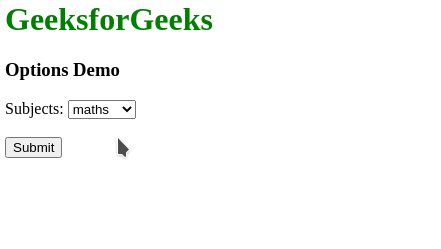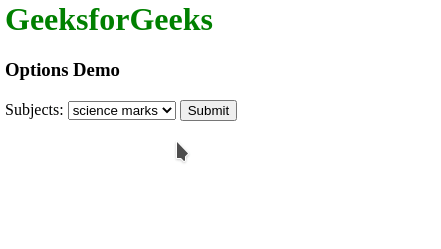Open In App

# How to define an option in a drop-down list in HTML5 ?

In this article, we will learn how to define an option in a drop-down list in HTML5. The <select> element is used to create a drop-down lists in HTML. This is generally used to present a list of options to the user in a form so that the user could choose one option as the needed one.

The <option> element which is defined inside the <select> element defines the options that are available in the drop-down list. This tag contains the following attributes:

The below examples show how an option can be defined in a drop-down list.

Example 1: In this example, the subjects are displayed as options in the drop-down list.

## HTML

 `<``html``>``<``body``>``  ``<``h1` `style``=``"color: green"``>``    ``GeeksforGeeks``  ````  ``<``h3``>Options Demo``    ``<``form``>``      ``<``label` `for``=``"sub"``>Subjects: ``      ```` ` `      ````      ``<``select` `name``=``"sub"` `id``=``"sub"``>`` ` `        ````        ``<``option` `value``=``"maths"``>``          ``maths``        ````        ``<``option` `value``=``"science"``>``          ``science``        ````        ``<``option` `value``=``"social"``>``          ``social``        ````        ``<``option` `value``=``"hindi"``>``          ``hindi``        ````      ````      ``<``br``><``br``>``      ``<``input` `type``=``"submit"` `value``=``"Submit"``>``  ```````

Output:Example 2: In this example, the marks of the subject are specified using the value attribute in the options of the drop-down list.

## HTML

 `<``html``>``<``body``>``  ``<``h1` `style``=``"color: green"``>``    ``GeeksforGeeks``  ````  ``<``h3``>Options Demo``  ``<``form``>``    ``<``label` `for``=``"sub"``>Subjects: ``    ```` ` `    ````    ``<``select` `name``=``"sub"` `id``=``"marks"``>`` ` `      ````      ``<``option` `value``=``"98"``>``        ``maths marks``      ````      ``<``option` `value``=``"90"``>``        ``science marks``      ````      ``<``option` `value``=``"90"``>``        ``social marks``      ````      ``<``option` `value``=``"45"``>``        ``hindi marks``      ````    ```` ` `    ``<``input` `type``=``"submit"` `           ``value``=``"Submit"``>``  ```````

Output: